Question

# PLEASE ANSWER BOTH, I KNOW ITS LONG BUT ITS FOR FUTURE GRADING. 6. Consider a binary...

6. Consider a binary Fe-C alloy with 1.5 wt.% C. At 1600 C (a) The phase present is ___________________________. (b) The composition of this phase is ______ wt. % C.

At 1400 C (c) The phases present are: ______________________ (phase A) and ___________________ (phase B). (d) The composition of phase A is _________ wt. % C and the composition of phase B is _________ wt.% C.

At 1100 C (e) The phase present is __________________________. (f) The composition of this phase is ______ wt. % C.

At 727.1 C (g) The phases present are: ______________________ (phase C) and ____________________________ (phase D). (h) The composition of phase C is _____ wt. % C and the composition of phase D is ____ wt.% C. (i) The amount of phase C is ______ wt. %. (j) Draw and label the microstructure observed at this temperature.

At 726.95 C (k) The phases present are: ______________________ (phase X) and _________________________ (phase Y). (l) The composition of phase X is _____ wt. % C and the composition of phase Y is ____ wt.% C. (m) The amount of phase X is ______ wt. %. (n) Draw and label the microstructure observed at this temperature. (o) The phase that is both in the proeutectoid microstructure and the eutectoid microstructure is __________________. ______ wt.% is in the proeutectoid microstructure.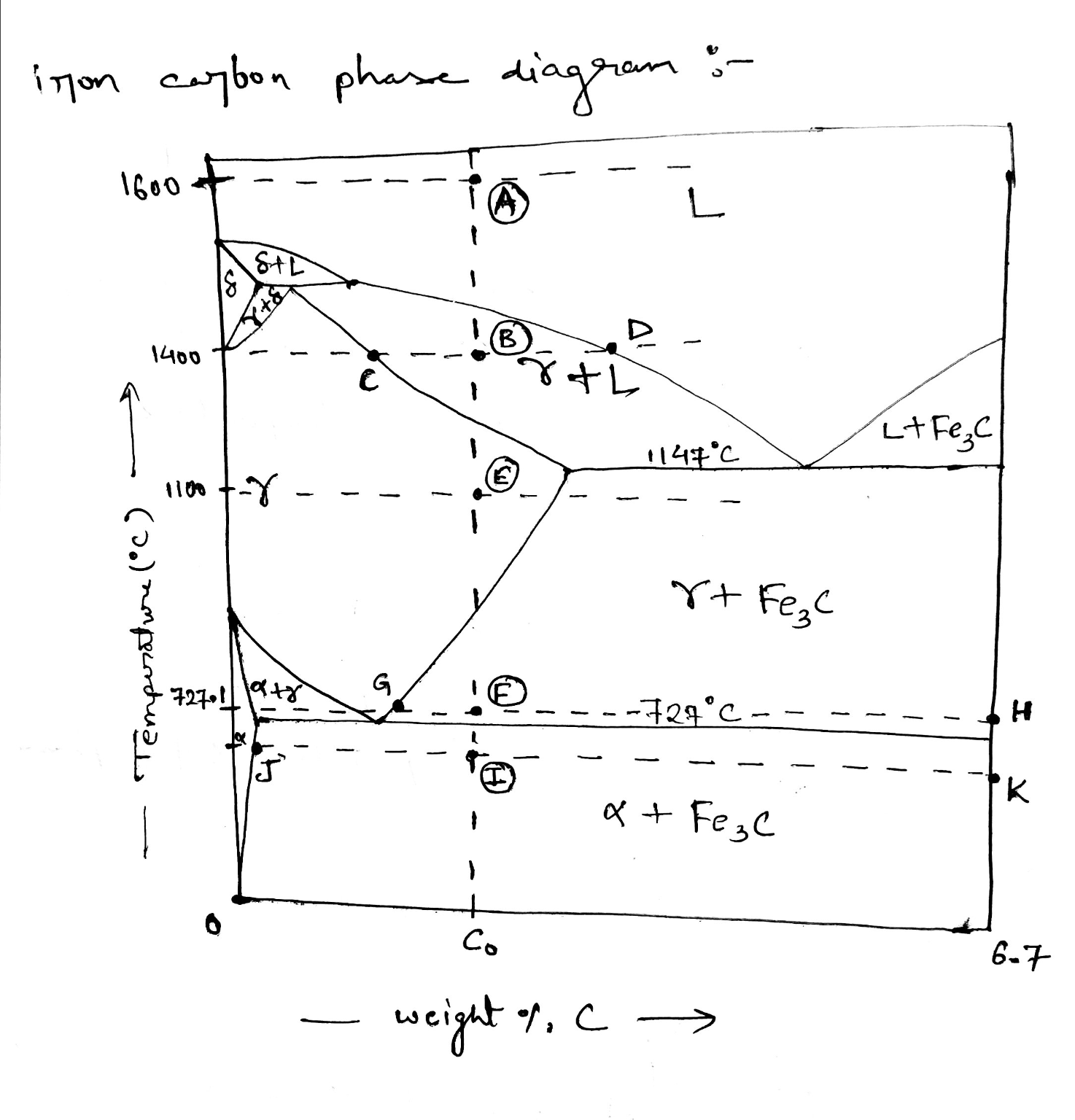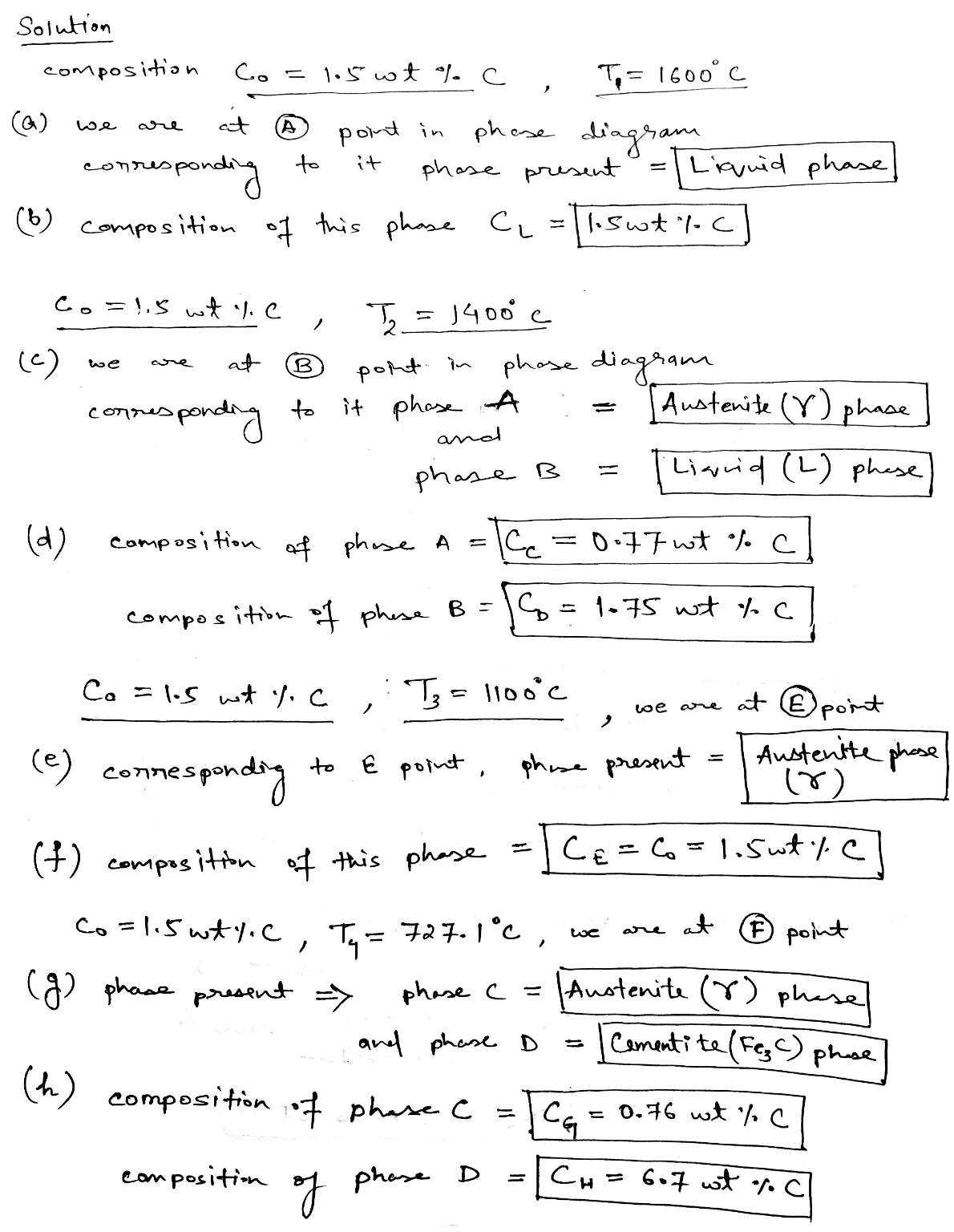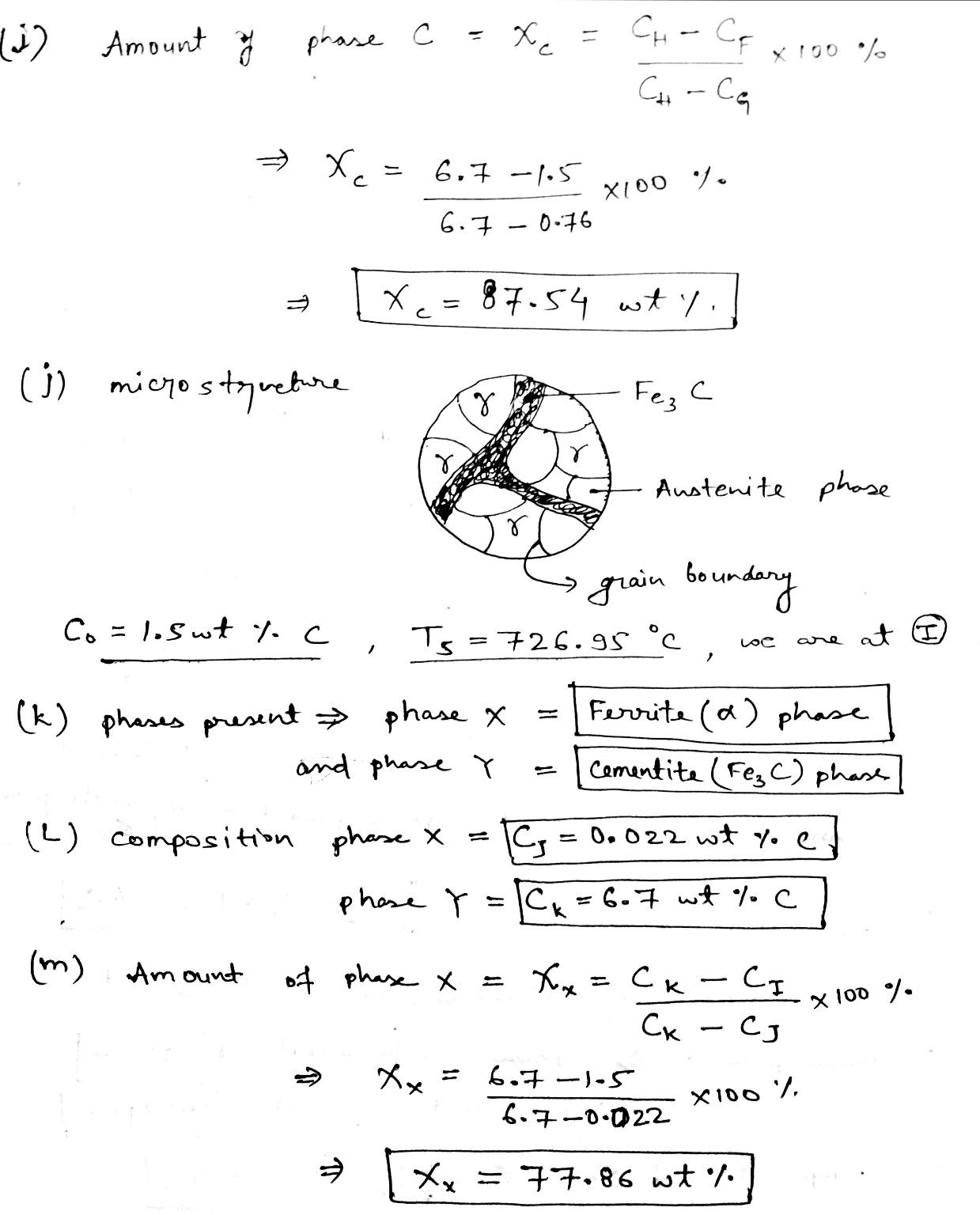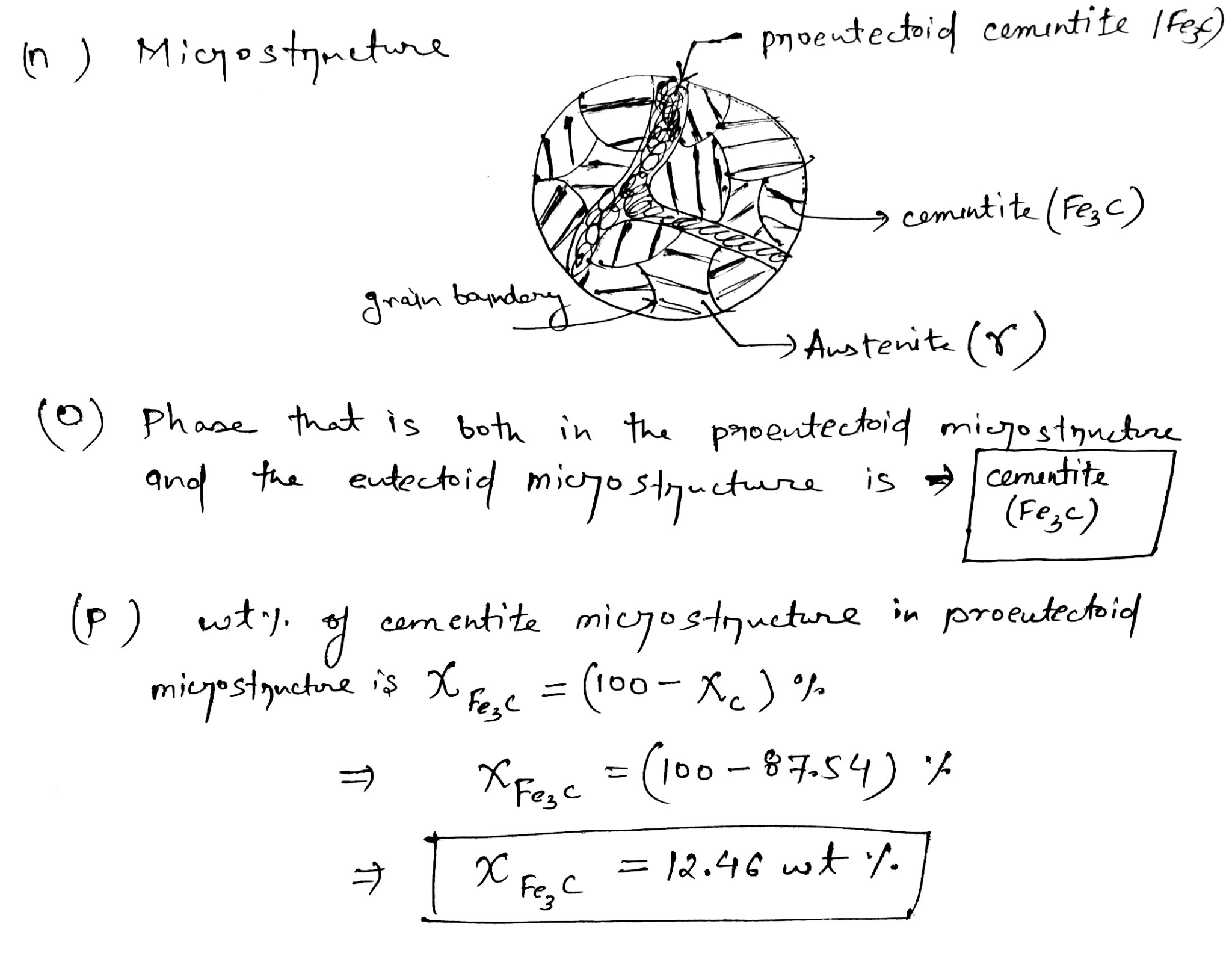#### Earn Coins

Coins can be redeemed for fabulous gifts.

Similar Homework Help Questions
• ### please answer question 2,3,4 ​​​​​​​ A critical feature of steel is that a considerable amount of...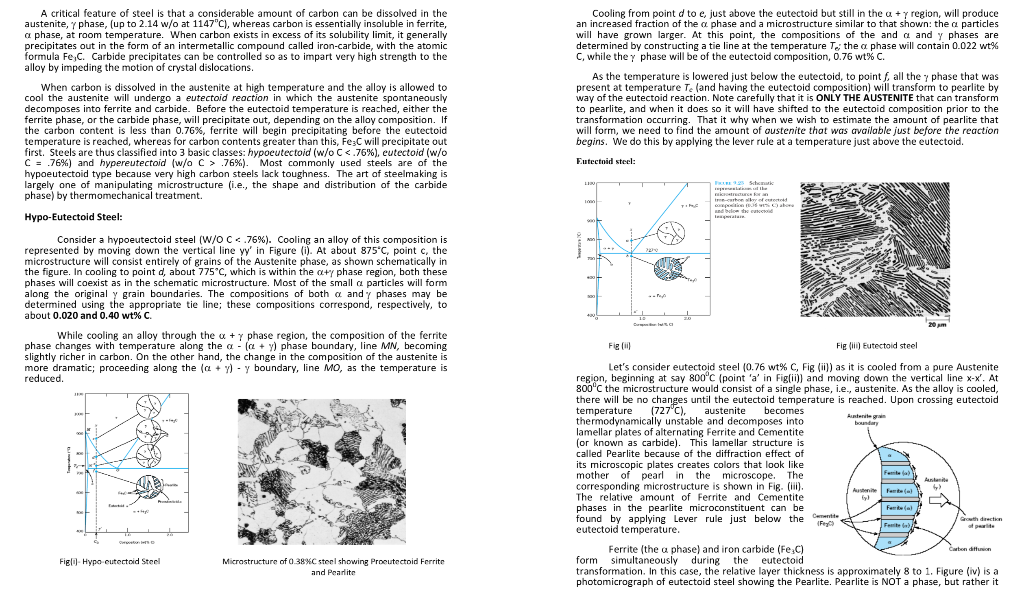please answer question 2,3,4 ​​​​​​​ A critical feature of steel is that a considerable amount of carbon can be dissolved in the austenite, phase, (up to 2.14 w/o at 1147"C), whereas carbon is essentially insoluble in ferrite, Cooling from point d to e, just above the eutectoid but still in the an increased fraction of the +y region, will produce phase and a microstructure similar to that shown: the particles precinitates out in the form af an intermetallic.compound called iron-carbide...

• ### Help with part 2 for c please! 800 727°C 0.76 0.022 a, Ferrite 600 a +...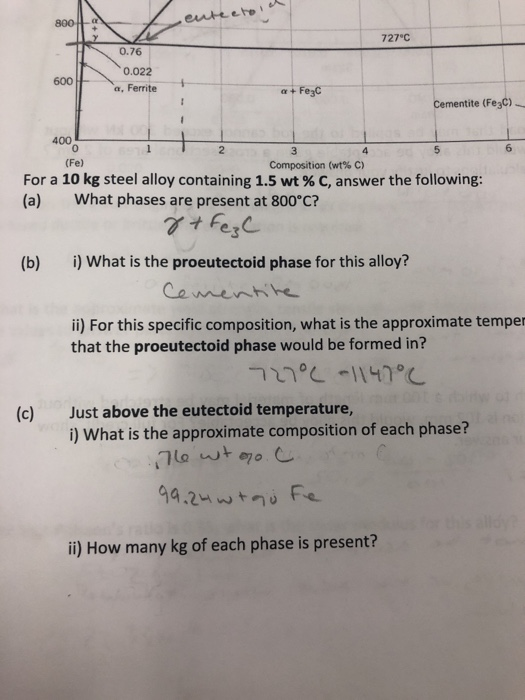Help with part 2 for c please! 800 727°C 0.76 0.022 a, Ferrite 600 a + Fe3C tite (Fe3C) 400 6 0 (Fe) 2 Composition (wt% C) For a 10 kg steel alloy containing 1.5 wt % C, answer the following: (a) What phases are present at 800°c? (b) i) What is the proeutectoid phase for this alloy? l) For this specific composition, what is the approximate temper that the proeutectoid phase would be formed in? Just above the eutectoid...

• ### 6. Consider the Ti-Ni system in the figure below. Atomic Percent Nickel 20 I800 1600 L...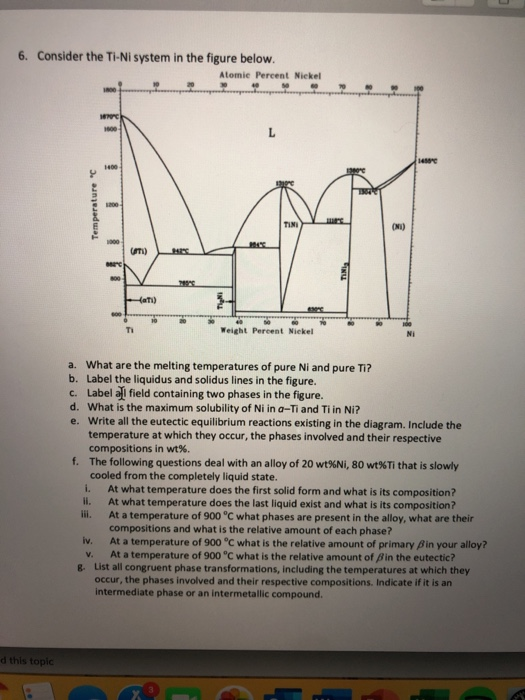6. Consider the Ti-Ni system in the figure below. Atomic Percent Nickel 20 I800 1600 L 1450C 1400 200 TIN (Ni) 1000 Un) re s00 Hati) s00 Ti Weight Percent Nickel N a. What are the melting temperatures of pure Ni and pure Ti? b. Label the liquidus and solidus lines in the figure. c. Label al field containing two phases in the figure. d. What is the maximum solubility of Ni in a-Ti and Ti in Ni? e. Write...

• ### In the Al-Cu phase diagram, determine what phases are present in the binary areas (white area). D...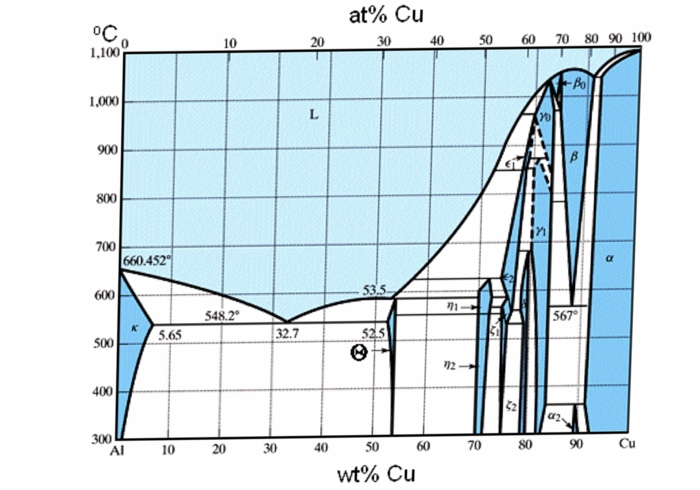In the Al-Cu phase diagram, determine what phases are present in the binary areas (white area). Determine all the phase transformations happened in the following phase diagram. For a 20 wt% Cu alloy at 500 oC, determine: The phases present The phase compositions The fraction of each phase Draw microstructure of this alloy at 700 oC, 560 oC and 300 oC. We are interested to design an alloy with heat treatable characteristics. Please suggest a composition range and explain why?...

• ### 15. From the iron-carbon phase diagram (see Figure below) a. What are the concentrations of carbon...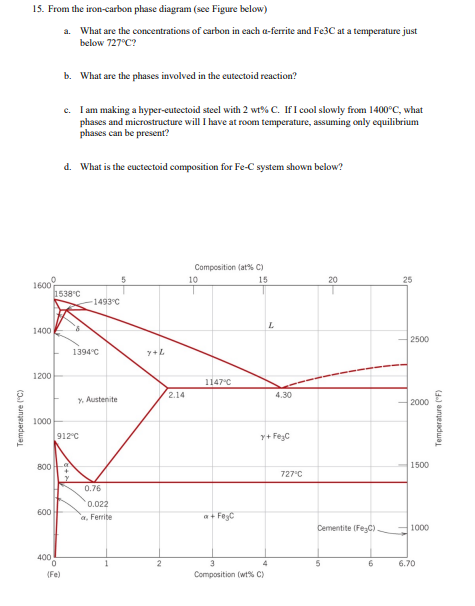15. From the iron-carbon phase diagram (see Figure below) a. What are the concentrations of carbon in each a-ferrite and Fe3C at a temperature just below 727°C? b. What are the phases involved in the eutectoid reaction? c. I am making a hyper-eutectoid steel with 2 wt%C. If I cool slowly from 1400°C, what phases and microstructure will I have at room temperature, assuming only equilibrium phases can be present? d. What is the euctectoid composition for Fe-C system shown...

• ### The microstructure of an iron-carbon alloy consists of proeutectoid cementite and pearlite; the mass fractions of...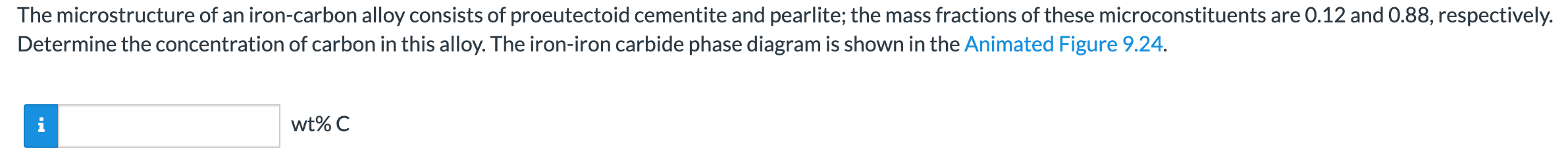The microstructure of an iron-carbon alloy consists of proeutectoid cementite and pearlite; the mass fractions of these microconstituents are 0.12 and 0.88, respectively. Determine the concentration of carbon in this alloy. The iron-iron carbide phase diagram is shown in the Animated Figure 9.24. wt%C Composition = 0 wt% C Composition = 0 at% C Temperature = 400 °C Temperature = 752 F 2025 Composition (at% C) 10 15 160025 1600 97538 9493 °C 1400 1400 2500 | 1394 °C y+L...

• ### would like the following introduction to materail science question answeres with comments (6) Below is a...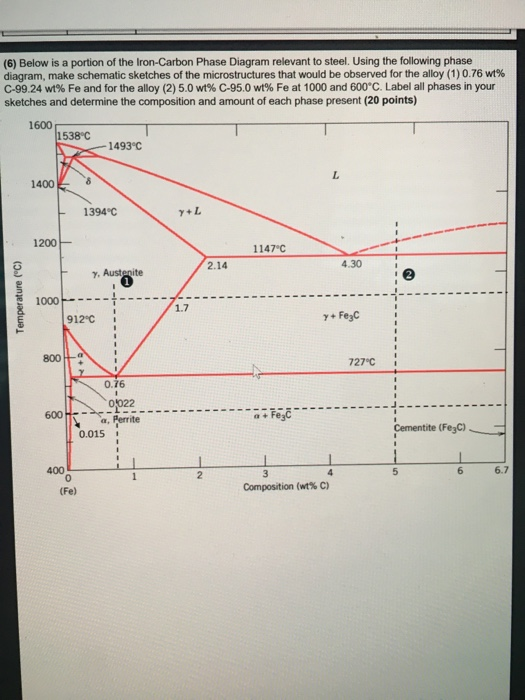would like the following introduction to materail science question answeres with comments (6) Below is a portion of the Iron-Carbon Phase Diagram relevant to steel. Using the following phase diagram, make schematic sketches of the microstructures that would be observed for the alloy (1) 0.76 wt% C-99.24 wt% Fe and for the alloy (2) 5.0 wt% C-95.0 wt% Fe at 1000 and 600°C. Label all phases in your sketches and determine the composition and amount of each phase present (20...

• ### The following is the phase diagram for the lead-tin alloy: Pb-Sn Phase Diagram Composition (at% Sn)...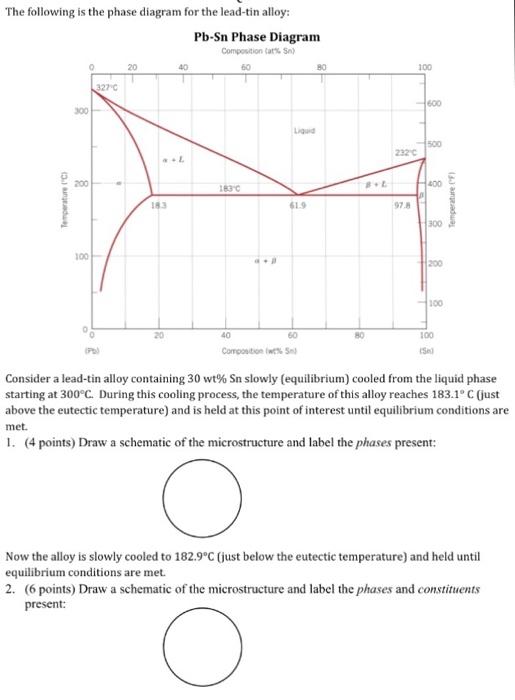The following is the phase diagram for the lead-tin alloy: Pb-Sn Phase Diagram Composition (at% Sn) 100 300 500 200 83 C 61.9 97.8 300 100 100 100 Consider a lead-tin alloy containing 30 wt% Sn slowly (equilibrium) cooled from the liquid phase starting at 300°C. During this cooling process, the temperature of this alloy reaches 183.1° C (just above the eutectic temperature) and is held at this point of interest until equilibrium conditions are met. 1. (4 points) Draw...

• ### For a 70 wt % You are given the binary eutectic copper-silver phase diagram shown in Figure 2. Cu...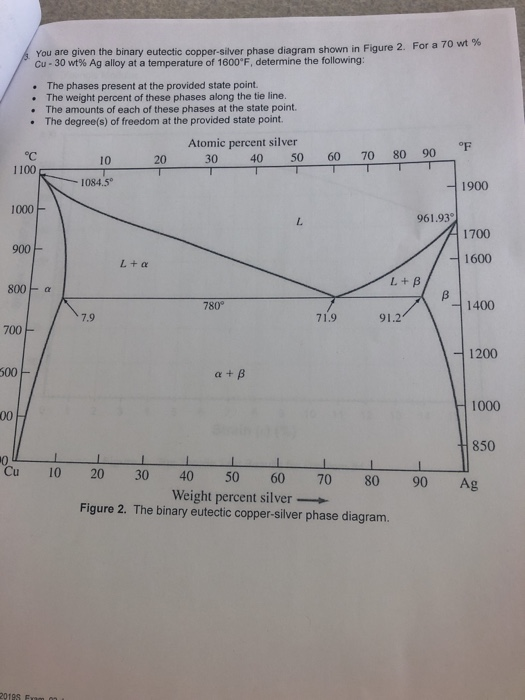For a 70 wt % You are given the binary eutectic copper-silver phase diagram shown in Figure 2. Cu-30 wt% Ag alloy at a temperature of 1600°F, determine the following: . The phases present at the provided state point The weight percent of these phases along the tie line. The amounts of each of these phases at the state point. The degree(s) of freedom at the provided state point Atomic percent silver 30 40 50 60 70 80 90 10...

• ### 8. For the Fe-C diagram answer the following a) Give the common name for the following...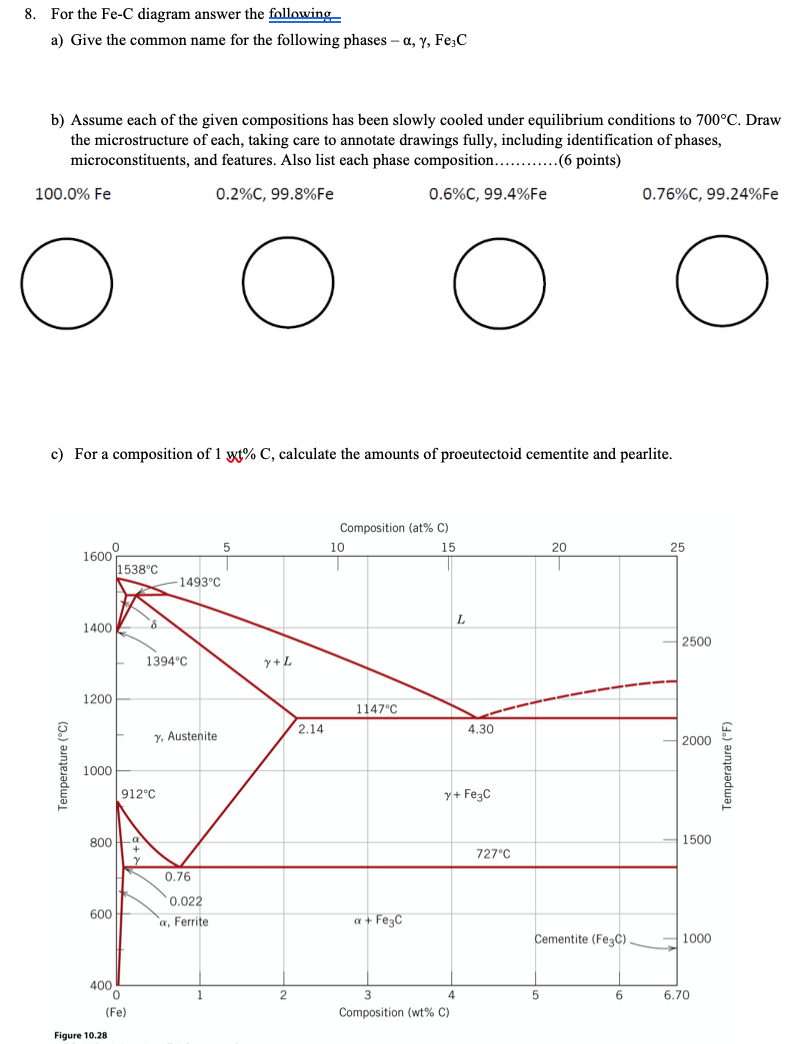8. For the Fe-C diagram answer the following a) Give the common name for the following phases - a, Y, FeC b) Assume each of the given compositions has been slowly cooled under equilibrium conditions to 700°C. Draw the microstructure of each, taking care to annotate drawings fully, including identification of phases, microconstituents, and features. Also list each phase composition...... (6 points) 100.0% Fe 0.2%C, 99.8%Fe 0.6%C, 99.4%Fe 0.76%C, 99.24%Fe () () () c) For a composition of 1 wt%...